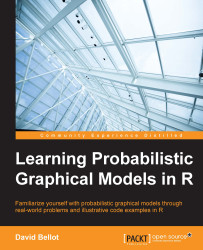•#### Learning Probabilistic Graphical Models in R#### Overview of this book

Probabilistic graphical models (PGM, also known as graphical models) are a marriage between probability theory and graph theory. Generally, PGMs use a graph-based representation. Two branches of graphical representations of distributions are commonly used, namely Bayesian networks and Markov networks. R has many packages to implement graphical models. We’ll start by showing you how to transform a classical statistical model into a modern PGM and then look at how to do exact inference in graphical models. Proceeding, we’ll introduce you to many modern R packages that will help you to perform inference on the models. We will then run a Bayesian linear regression and you’ll see the advantage of going probabilistic when you want to do prediction. Next, you’ll master using R packages and implementing its techniques. Finally, you’ll be presented with machine learning applications that have a direct impact in many fields. Here, we’ll cover clustering and the discovery of hidden information in big data, as well as two important methods, PCA and ICA, to reduce the size of big problems.
Learning Probabilistic Graphical Models in RCreditswww.PacktPub.comPrefaceFree Chapter
Probabilistic ReasoningExact InferenceLearning ParametersBayesian Modeling – Basic ModelsApproximate InferenceBayesian Modeling – Linear ModelsProbabilistic Mixture ModelsAppendixIndex## Summary

In this chapter, we saw the standard linear model. This model is one of the most important models in statistics and provides a simple, additive way to represent relationships between observed variables and a target.

Estimating good parameters for a linear model can be hard sometimes and one should be very careful not to trust the results immediately. However, a Bayesian approach to the problem helps to include prior knowledge into the model and drive it toward a more stable and usable solution.

We saw ridge regression and Bayesian linear regression. We saw that, when the parameters have a Gaussian prior, then these two approaches are equivalent and very easy to compute.

Using a simple example, we saw that a standard regression can lead to completely over-fitted results and that the Bayesian approach solved the problem.

In the next chapter, we will look at more advanced models for dealing with clusters of data, called mixture models. These models make the assumption that the data is generated...